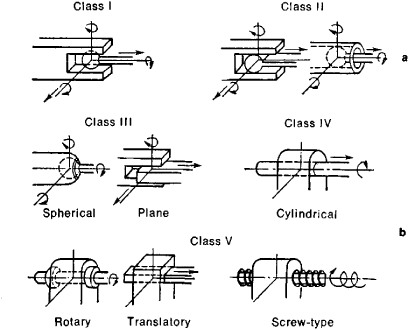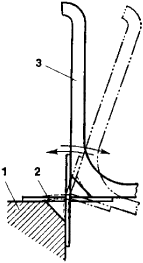# Kinematic Pair

(redirected from Higher kinematic pair)
The following article is from The Great Soviet Encyclopedia (1979). It might be outdated or ideologically biased.

## Kinematic Pair

a movable coupling of two rigid members that imposes restraints on the relative motion of the members by the conditions of linkage. Each of the conditions of linkage removes one degree of freedom—that is, the possibility of one of the six independent relative motions in space. Three translational motions (in the direction of the three coordinate axes) and three rotational motions (around the axes) are possible in the rectangular system of coordinates.Figure 1. Kinematic pairs: (a) higher, (b) lower

Kinematic pairs are divided into five classes according to the number of conditions of linkage S imposed by the linkage. The number of degrees of freedom of a kinematic pair W = 6 — S. Within each class kinematic pairs are subdivided into types according to the relative motions of the members that remain possible. Kinematic pairs are also classified according to the type of contact between the members as lower kinematic pairs (with surface contact) and higher kinematic pairs (with line or point contact). Higher kinematic pairs are possible in all five classes and for many types; lower pairs can be only of three classes and six types (Figure 1).Figure 2. Diagram of a play-free hinge: (1) fixed part, (2) deformable elements (flat springs), (3) lever

A distinction is also made between geometrically closed and open kinematic pairs. In geometrically closed pairs, constant contact of the surfaces is assured by the shape of components (for example, in all kinematic pairs shown in Figure 1); in open pairs, a compressive force is required for closure (for example, in a cam mechanism). Some movable couplings that have several intermediate rolling bodies (such as ball bearings and roller bearings) are also conventionally considered to be kinematic pairs; this is also true of junctions with deformable intermediate components (for example, play-free hinges of devices using flat springs; Figure 2).

N. IA. NIBERG